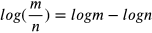1
52
views
34b
Problem

Problem 34b

Chapter 1: Functions and ModelsTextbook ExpertVerified Tutor
13 Oct 2021

Step-by-step explanation

Step 1.

logarithm which has a fixed base of 10 is called the common logarithm or decimal logarithm this is also called as briggsian logarithm because it is introduced by mathematician henry briggs. if m is a number then it is denoted as example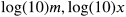or simply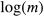.

properties of common logarithm is

1. product rule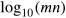=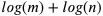2. power rule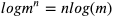3. division rule